# Powers

Is true for any number a,b,c,d equality:?

$\left(a-b-c-d{\right)}^{6}=\left(d+c+b-a{\right)}^{6}$

Result

### Step-by-step explanation: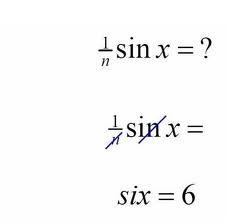Did you find an error or inaccuracy? Feel free to write us. Thank you!## Related math problems and questions:

• Comparing powers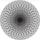How many times is number 56 larger than number 46?
• Square Number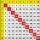If to a square of integer number add 17, we get the square of the next integer number. What is the original number?
• Binomials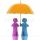To binomial 36x2-168x add a number to the resulting trinomial be square of binomial.
• Comparing exponents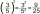A) 8 (6²)(8²) b) 16 (6²)(8²) c) (6¹+²) (8²)
• PowerNumber ?. Find the value of x.
• Square 2Points D[10,-8] and B[4,5] are opposed vertices of the square ABCD. Calculate area of the square ABCD.
• The ticketsThe tickets to the show cost some integer number greater than 1. Also, the sum of the price of the children's and adult tickets, as well as their product, was the power of the prime number. Find all possible ticket prices.
• Square roots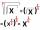What is equal to the product of the square roots of 295936?
• Simplest form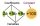Find the simplest form of the following expression: 3 to the 2nd power - 1/4 to the 2nd power.
• Primes 2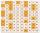For what primes p,q,r is true: p2-(q+r)2=647
• Square area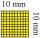Complete the table and then draw each square. Provide exact lengths. Describe any problems you have. Side Length Area Square 1 1 unit2 Square 2 2 units2 Square 3 4 units2
• PowersExpress the expression ? as the n-th power of the base 10.
• RootThe root of the equation (x-19)2 -10 = x2 -11x is (equal or greater or less than zero)? ...
• Digits A, B, CFor the various digits A, B, C is true: the square root of the BC is equal to the A and sum B+C is equal to A. Calculate A + 2B + 3C. (BC is a two-digit number, not a product).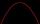Solve pure quadratic equation -5x2 +10 = 0.Volume of the cube is 216 cm3, calculate its surface area.What is the length x of the chord circle of diameter 115 m if the distance from the center circle is 11 m?